## Plotting Bézier triangular patches with Python Plotly

### The de Casteljau algorithm for computing points on a triangular Bézier patch

A Bézier triangular patch is a parameterized polynomial surface. Its parameters belong to a triangular 2D region, $T$. The triangle vertices, $T_0, T_1, T_2$, are denoted counterclockwise like in the figure below:

In :
from IPython.display import Image
Image(filename='Imag/triangle.png')

Out: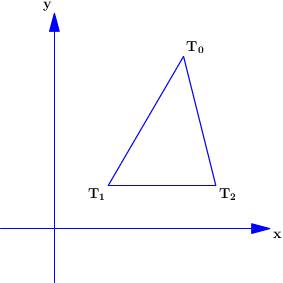Each point $P$ in $T$ is expressed as a convex combination of the vertices $T_0, T_1, T_2$:

$P=\lambda_0T_0+\lambda_1T_1+\lambda_2T_2$, $\lambda_i\geq 0$, $i=\overline{0,2}$, and $\lambda_0+\lambda_1+\lambda_2=1$

The scalars $\lambda_i$ are the barycentric coordinates of the point $P$.

If $(x_i, y_i)$ are the cartesian coordinates of the vertices $T_i$, $i=\overline{0,2}$, and $P(x, y)$ is a point in the corresponding triangular region, then its baycentric coordinates are given by the solution of the linear system:

$$\left[\begin{array}{ccc} x_0&x_1&x_2\\ y_0&y_1&y_2\\ 1&1&1\end{array}\right] \left[\begin{array}{c}\lambda_0\\\lambda_1\\\lambda_2\end{array}\right]= \left[\begin{array}{c} x\\y\\1\end{array}\right]$$

There is a one-to-one correspondence between the points of the triangle $T$ and the triangle $\Delta$, in 3D, of vertices $A_0(1,0,0)$, $A_1(0,1,0)$, $A_2(0,0,1)$. Namely, to each $P(x,y)\in T$ one associates its barycentric coordinates $(\lambda_0, \lambda_1, \lambda_2)\in\Delta$.

In :
Image(filename='Imag/triangle3D.png')

Out: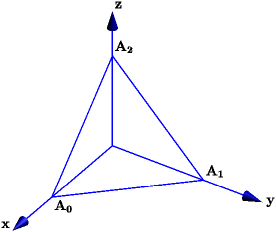A Bézier triangular patch is defined by an integer $n>0$, and a triangular net of 3D points ${\bf b}_{ijk}$, with $i, j, k\geq 0$ integers such that $i+j+k=n$. Its parameterization is: $S: (\lambda_0, \lambda_1, \lambda_2)\in \Delta \to \sum_{i+j+k=n}\frac{n!}{i!j!k!}\lambda_0^i\lambda_1^j\lambda_2^k {\bf b}_{ijk}$

Since the sum of powers of $\lambda_0, \lambda_1, \lambda_2$ is $n$, the patch is said to have the total degree $n$.

A point on such a surface corresponding to the parameters $(\lambda_0, \lambda_1, \lambda_2)$ is computed recursively by the triangular de Casteljau algorithm:

$$\begin{array}{lll} {\bf b}_{ijk}^0&=&{\bf b}_{ijk}\\ {\bf b}_{ijk}^r&=&\lambda_0{\bf b}_{i+1\,j\,k}^{r-1}+\lambda_1{\bf b}_{i\, j+1\, k}^{r-1} +\lambda_2{\bf b}_{i\,j\,k+1}^{r-1},\end{array}$$

where $r=\overline{1,n}$, and $i+j+k=n-r$.

The last computed point, ${\bf }b_{000}^n$, is a point on the surface.

Details on the triangular Bézier patches can be found in:

1. G Farin, Curves and Surfaces for Computer Aided Geometric Design: A Practical Guide, Morgan Kaufmann, 2002.
2. J. Gallier, Curves and Surfaces in Geometric Modeling: Theory and Algorithms, Morgan Kaufmann, 1999. A free electronic version can be downloaded here.

The $(n+1)(n+2)/2$ control points, ${\bf b}_{ ijk}$, are placed in a triangular structure in the same way as the points $(i/n, j/n, k/n)$ are located in the triangle $\Delta$ of vertices $A_0(1,0,0)$, $A_1(0,1,0)$, $A_2(0,0,1)$.

We illustrate here the case $n=3$:

In :
Image(filename='Imag/ijk.png')

Out: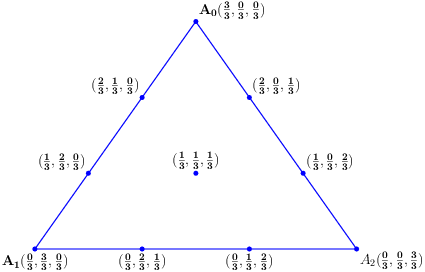Hence to generate a Bézier triangular patch of total degree $3$ we start with a net of points:

$$\begin{array}{lllllll} & & & {\bf b}_{300} & & & \\ & & & & & & \\ & & {\bf b}_{210} & & {\bf b}_{201} & & \\ & & & & & & \\ & {\bf b}_{120} & & {\bf b}_{111} & & {\bf b}_{102} & \\ & & & & & & \\ {\bf b}_{030} & & {\bf b}_{021}& & {\bf b}_{012} & & {\bf b}_{003} \end{array}$$

The point indices in a triangular net or the indices that define points in a triangular grid of $\Delta$, as above, are returned by the following function::

In :
def indexes(n):
return [(i,j, n-(i+j)) for i in range(n,-1, -1) for j in range(n-i, -1, -1)]

In :
print indexes(3)

[(3, 0, 0), (2, 1, 0), (2, 0, 1), (1, 2, 0), (1, 1, 1), (1, 0, 2), (0, 3, 0), (0, 2, 1), (0, 1, 2), (0, 0, 3)]


In each step, $r$, of the de Casteljau recursive formula, one computes succesively the convex combinations with the same coefficients, $\lambda_0, \lambda_1, \lambda_2$, of all triplets of nearby points in the net ${\bf b}^{r-1}_{ijk}$. The relative position of points in a such triplet is illustrated in the next cell:

$$\begin{array}{lll} & *& \\ *& &*\end{array}$$

In the following we give the nets of points resulted from each step $r$. Starting with the initial control points:

$$\begin{array}{lllllll} & & & {\bf b}^0_{300} & & & \\ & & & & & & \\ & & {\bf b}^0_{210} & & {\bf b}^0_{201} & & \\ & & & & & & \\ & {\bf b}^0_{120} & & {\bf b}^0_{111} & & {\bf b}^0_{102} & \\ & & & & & & \\ {\bf b}^0_{030} & & {\bf b}^0_{021}& & {\bf b}^0_{012} & & {\bf b}^0_{003} \end{array}$$

the step $r=1$ computes the points:

$$\begin{array}{lllll} & & {\bf b}^1_{300} & & \\ & & & & \\ & {\bf b}^1_{210} & & {\bf b}^1_{201} & \\ & & & & \\ {\bf b}^1_{120} & & {\bf b}^1_{111} & & {\bf b}^1_{102} & \end{array}$$

while $r=2$, the points:

$$\begin{array}{lll} & {\bf b}^2_{300} & \\ & & \\ {\bf b}^2_{210} & & {\bf b}^2_{201} \end{array}$$

In the last step, $r=3$, we get the point on the surface, ${\bf b}^3_{300}$, corresponding to the parameters $(\lambda_0, \lambda_1,\lambda_2)$.

To implement the de Casteljau algorithm we store the control points in a list of points, concatenating the rows of the theoretical triangular net.

We define the function deCasteljau_step that evaluates the convex combinations for one step of the de Casteljau formula. Its arguments are the degree n, the list of points, b, and the triplet of barycentric coordinates, lam:

In :
import numpy as np
from __future__ import division

In :
def deCasteljau_step(n, b, lam):

i=0
j=1
for nr in range(1, n+1):
for k in range(nr):
b[i]=lam*b[i]+lam*b[j]+lam*b[j+1]
i+=1
j+=1
j+=1
return b[:-(n+1)]


de Casteljau algorithm is implemented by the following recursive function:

In :
def deCasteljau(n,b,lam):

if len(b)>1:
return deCasteljau(n-1, deCasteljau_step(n, b, lam), lam)
else:
return b


### A discretization method of a triangular Bézier patch for plotting with Plotly

In the following we present a method of discretization of a triangular Bézier patch, such that to get the data that define a plotly Surface.

The basic idea is to define a rectangular grid that covers the triangle $\Delta$ of barycentric coordinates. The deCasteljau function, evaluated at each point $(\lambda_0, \lambda_1, \lambda_2)$ of this grid, defines a triangular net of points on the surface. These points are then integrated into a meshgrid over the rectangle [min(X), max(X)], [min(Y), max(Y)], where X and Y are the lists of x, respectively y-coordinates of the points on the surface.

We start with a triangular grid of the triangle $\Delta$ constructed from indexes returned by the function indexes, called for an even integer $2m$.

We illustrate our method for a low value of $m=4$ (see the next image).

In :
Image(filename='Imag/triangulation.png')

Out: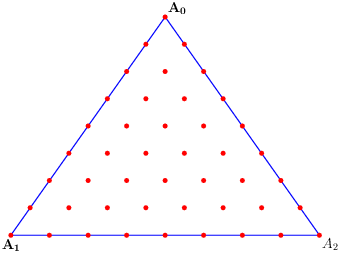On each side we have $2m+1$ points. Considering the points on the side $A_1A_2$ as reference points, we exclude from this grid the points that do not lie on perpendiculars at reference points, on $A_1A_2$. That is, we remove the points in the odd rows (parallel to $A_1A_2$).

In fact we define a function even_rows that just generate only the points in the even rows of the above triangulation:

In :
def even_row_pts(p):#p=2*m

if p%2:
raise ValueError('p must be an even integer')
I=[2*k for k in range (p+1)]
return [(i/p,j/p, 1-(i+j)/p) for i in I[::-1] for j in range(p-i, -1, -1)]


and get a less dense grid:

In :
Image(filename='Imag/even_rows.png')

Out: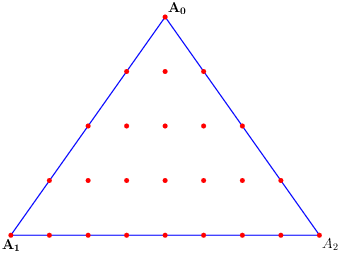Now to each two consecutive points placed on the same perpendicular to $A_1A_2$ one associates the mid point. Thus we construct additional points to the initial grid (the green points in the next image):

In :
def additional_weights(m, weights):

wei=map(np.array, weights)
new_weights=[]
a=0
for k in range(1, m+1):
for j in range(1,2*k):
new_weights.append((wei[a]+wei[a+2*k])/2)
a=a+1
return list(map(tuple, new_weights))


The function grid mixes the two lists of points:

In :
def grid(m, weights, new_weights):

L=len(weights)
barycenters=[]
for  k in range(m):
L=2*k+1
enD=k**2+L
barycenters +=weights[k**2: enD] +new_weights[k**2: enD]
barycenters+=weights[m**2:]
return barycenters


and the final grid of the triangle $A_0A_1A_2$ looks as follows:

In :
Image(filename='Imag/finalgrid.png')

Out: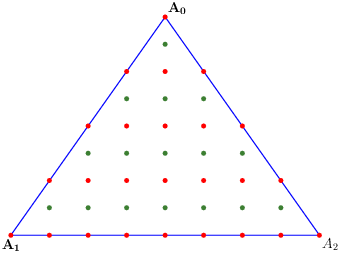To each point (triplet of weights) in this grid one associates a point on the Bézier patch.

The following function discretizes a Bézier surface of degree n, control points b, and grid points barycenters, defined above:

In :
def surface_points(n, b, barycenters):

points=[]
for weight in barycenters:
b_aux=np.array(b)
points.append(deCasteljau(n, b_aux, weight))
return zip(*points)


In order to plot the discretized patch we need to construct three arays of shape (2m+1,2m+1) (or a list of lists) that contain in an appropriate position the x, y, respectively z-coordinates of the computed points.

The values in a list of coordinates are placed succesively in the red positions, while outside the triangle, similar to the triangle $A_1A_1A_2$, we store None values:

In :
Image(filename='Imag/gridData.png')

Out: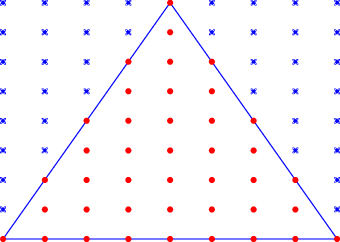The function move_data_to_triangle returns a list of lists. The values in a list of coordinates, D, are assigned correspondingly to locations placed within the formal triangle included in this list of lists:

In :
def move_data_to_triangle(m, D):

DD=[]
pos=0
for i in range(m):
L1=[None]*(2*m+1)
L2=[None]*(2*m+1)
idx=[m-i+J for J in range(2*i+1)]

for k in range(2*i+1):
L1[idx[k]]=D[pos]
pos+=1
for k in range(2*i+1):
L2[idx[k]]=D[pos]
pos+=1

DD.append(L1)
DD.append(L2)
K=-(2*m+1)
DD.append(D[K:])
return DD



set_data_for_Surface includes all previous functions that prepare data for a plot:

In :
def set_data_for_Surface(n, b, m):

if len(b)!=(n+1)*(n+2)/2:
raise ValueError('incorect number of control points')
weights=even_row_pts(2*m)
grid_pts=grid(m, weights, new_weights)
X, Y, Z=surface_points(n, b, grid_pts)
ZZ=move_data_to_triangle(m, Z)
XX= move_data_to_triangle(m, X)
YY=move_data_to_triangle(m, Y)
return [XX, YY, ZZ]


A colorscale derived from the future viridis colormap is used to plot a Surface:

In :
viridisCS=[[0.0, '#440154'],
[0.06274509803921569, '#48186a'],
[0.12549019607843137, '#472d7b'],
[0.18823529411764706, '#424086'],
[0.25098039215686274, '#3b528b'],
[0.3137254901960784, '#33638d'],
[0.3764705882352941, '#2c728e'],
[0.4392156862745098, '#26828e'],
[0.5019607843137255, '#21918c'],
[0.5647058823529412, '#1fa088'],
[0.6274509803921569, '#28ae80'],
[0.6901960784313725, '#3fbc73'],
[0.7529411764705882, '#5ec962'],
[0.8156862745098039, '#84d44b'],
[0.9411764705882353, '#d8e219'],
[1.0, '#fde725']]

In :
import plotly.plotly as py
import plotly.tools as tls
from plotly.graph_objs import *

In :
py.sign_in('empet', 'my_api_key')


plot_Surface generates a triangular Bézier patch of degree n and control points b, over the triangular grid of $2m+1$ points on each side of the barycenter triangle $\Delta$:

In :
def plot_Surface(n, b, m,  plot_title):

x, y, z= set_data_for_Surface(n, b, m)

trace = Surface(
z=z,
x=x,
y=y,
colorscale=viridisCS
)

axis = dict(
showbackground=True,
backgroundcolor="rgb(230, 230,230)",
gridcolor="rgb(255, 255, 255)",
zerolinecolor="rgb(255, 255, 255)",
)

layout = Layout(
title=plot_title,
width=600,
height=600,
scene=Scene(
xaxis=XAxis(axis),
yaxis=YAxis(axis),
zaxis=ZAxis(axis)
)
)
fig = Figure(data=[trace], layout=layout)
return fig


Finally we generate our first Bézier patch of degree $n=4$, over a grid corresponding to $m=80$:

In :
n=4
b=[[2.0, 3.0, 2.0],
[1.5, 2.25, 3.0],
[2.5, 2.25, 5.0],
[1.0, 1.5, 4.0],
[2.0, 1.5, 5.0],
[3.0, 1.5, 3.0],
[0.5, 0.75, 1.0],
[1.5, 0.75, 3.0],
[2.5, 0.75, 1.0],
[3.5, 0.75, 4.0],
[0.0, 0.0, 2.0],
[1.0, 0.0, 5.0],
[2.0, 0.0, 3.0],
[3.0, 0.0, 1.0],
[4.0, 0.0, 4.0]]
m=80

In :
fig=plot_Surface(n, b, m,  'Bezier triangular patch of degree 4')
py.iplot(fig, filename='Bezier-triangular-patch')

Out:

### Plotting the control polyhedron of a triangular Bézier patch

A Bézier triangular patch of degree $n$ and control points ${\bf b}_{ijk}$ interpolates the corners of the triangular net formed by these points, that is, it interpolates the points ${\bf b}_{n00}, {\bf b}_{0n0}, {\bf b}_{00n}$, and it is included in the convex hull of all its control points.

To illustrate this property we define the control polyhedron of the patch, i.e. the plotly trace we get connecting with lines the points in a triplet of nearby points.

The function triangles returns the lists of all triplets of nearby points in the triangular net ${\bf b}_{ijk}$, $i+j+k=n$:

In :
def triangles(n, b):

triplets=[]
i=0
j=1
for nr in range(1,n+1):
for k in range(nr):
triplets.append([b[i], b[j], b[j+1]])
i+=1
j+=1
j+=1
return triplets


and the function triangle_lines prepares data for a Scater3d trace of the control polyhedron edges:

In :
def triangle_lines(triplets):

line_pts=[]
for tri in triplets:
x, y, z=zip(*tri)
x=list(x)+[x]
y=list(y)+[y]
z=list(z)+[z]
line_pts.append(Scatter3d(x=x, y=y, z=z,
name='',
mode='lines+markers',
line=Line(color='#000762',
width=4),
marker=Marker(
symbol='dot',
size=5,
color='#000762')
)
)
return line_pts


The function plot_surface_polyedron plots both a Bézier patch and its control polyedron:

In :
def plot_surface_polyhedron(n, b, m, plot_title):

x, y, z= set_data_for_Surface(n, b, m)

trace = Surface(
z=z,
x=x,
y=y,
colorscale=viridisCS
)

triplets=triangles(n,b)
lines_pts=triangle_lines(triplets)

axis = dict(
showbackground=True,
backgroundcolor="rgb(230, 230,230)",
gridcolor="rgb(255, 255, 255)",
zerolinecolor="rgb(255, 255, 255)",
)

layout = Layout(
title=plot_title,
width=600,
height=600,
showlegend=False,
scene=Scene(
xaxis=XAxis(axis),
yaxis=YAxis(axis),
zaxis=ZAxis(axis)
)
)
fig = Figure(data=[trace]+lines_pts, layout=layout)
return fig


Data for a cubic (degree $n=3$) triangular Bézier patch and its control polyhedron:

In :
n=3
d=[[2.5, 2.5, 1.5],
[1.3, 2, 3],
[3, 2.5, 3],
[1, 1, 2],
[2.5, 1, 4],
[4, 1, 1],
[0, 1, 1],
[2, 0, 2],
[3, -0.5, 1.5],
[5, 0, -0.5]]
m=80

In :
fig=plot_surface_polyhedron(n,d, m, 'A cubic triangular Bezier patch and its control polyhedron')
py.iplot(fig, filename='Bezier-cubic')

Out:

### Triangular Bézier graphs and their contour plots

A degree $n$ triangular Bézier patch is the graph of a function defined on a planar triangular region of vertices $T_0, T_1, T_2$, if its control points have the coordinates ${\bf b}_{ijk}=(x_{ijk},y_{ijk}, z_{ijk})$, $i+j+k=n$, where $(x_{ijk},y_{ijk})$ are points in the triangular region $T$, of barycentric coordinates $(i/n, j/n, k/n)$, and $z_{ijk}$ are real values set by the user.

Initial data for defining such a graph are:

• the degree, $n$
• the triangle $T$ given as a list of three numpy arrays of shape (2,)
• a list, b_z, of $(n+1)(n+2)/2$ real values representing $z$-coordinates of the control points

The function:

In :
cartesian_coords=lambda w, T: w*T+w*T+w*T


calculates the cartesian coordinates of a point having barycentic coordinates $(w_0, w_1, w_2)$, with respect to a triangle $T$.

The control points of the graph are returned by the following function:

In :
def control_points(n, T, b_z):
ind=indexes(n)
weights=[(i/n, j/n, k/n) for (i, j, k) in ind]
b_xy=[cartesian_coords(w,T) for w in weights]
b_xy=map(list, b_xy)
len_bxy=len(b_xy)
b=[b_xy[k]+[b_z[k]] for k in range(len_bxy)]
return b


Let us define a Bézier triangular graph of degree $n=4$, over the triangle $T$:

In :
n=4
m=80
nr_ctrl_pts=(n+1)*(n+2)/2
T=[np.array([0, 1.5]), np.array([-1,0]), np.array([1, 0])]


$z$-coordinates of the control points are set arbitrary in the interval $[1,5]$:

In :
b_z=1+4*np.random.random(nr_ctrl_pts)


Then the control points of our graph are:

In :
b=control_points(n, T, b_z)

In :
fig=plot_Surface(n, b, m,  'Graph as a triangular Bezier patch')
py.iplot(fig, filename='triangular-Bezier-graph4')

Out:

### Contour plot of a triangular Bézier graph

Having a method to generate a triangular Bézier graph, we can generate its contour plot with Plotly.

We set the data for a plotly Contour, namely a 2D array or a list of lists for z-values on the surface, and two 1D lists for x, respectively y-coordinates of the grid points in the triangle $T$.

In :
n=4
m=80

In :
weights=even_row_pts(2*m)
grid_pts=grid(m, weights, new_weights)
X, Y, Z=surface_points(n, b, grid_pts)
ZZ=move_data_to_triangle(m, Z)[::-1]


Get the x-list for Contour:

In :
K=-(2*m+1)
XX= [cartesian_coords(w,T) for w in grid_pts[K:]]
x=sorted(list(set(XX)))


and the y-list:

In :
I=[k**2 for k in range(m)]
Lam=[]
for k in range(m):
Lam.append(weights[I[k]+k])
Lam.append(new_weights[I[k]+k])
Lam.append(weights[-(m+1)] )
YY=[cartesian_coords(w,T) for w in  Lam]
y=sorted(list(set(YY)))


Plot the triangular contour:

In :
title="Contourplot of a triangular Bezier function"
data = Data([
Contour(
z=ZZ,
x=x,
y=y,
autocontour=False,
zauto=False,
contours=Contours(
showlines=False,
start =min(Z),
end=max(Z),
size=0.1
),
colorscale=viridisCS,
),
])
layout = Layout(
title= title,
font= Font(family='Georgia, serif',  color='#635F5D'),
showlegend=False,
autosize=False,
width=500,
height=500,
xaxis=XAxis(
range=[min(x), max(x)],
showgrid=False,
),
yaxis=YAxis(
range=[min(y), max(y)],
showgrid=False,
),
margin=Margin(
l=40,
r=40,
b=85,
t=100,
autoexpand=True
),
)
fig = Figure(data=data, layout=layout)
py.iplot(fig, filename='Contourplot-triangular-Bezier-graph4')

Out:

Another pair graph-contour plot can be seen here:

In :
from IPython.core.display import HTML
def  css_styling():